# Constant-pressure Monte Carlo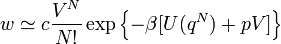$w \simeq c \frac{V^N}{N!} \exp \left\{ -\beta [ U(q^N) +pV ] \right\}$
for a change in volume,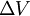$\Delta V$, one has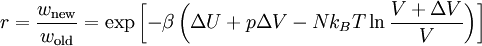$r = \frac{w_{\rm new}}{w_{\rm old}} = \exp \left[ -\beta \left( \Delta U + p\Delta V - Nk_BT \ln \frac{V+\Delta V}{V}\right)\right]$
If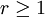$r \ge 1$ then the move is accepted, and if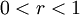$0 < r < 1$ then r is compared with a random number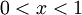$0 < x < 1$. If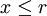$x \le r$ then the move is accepted.